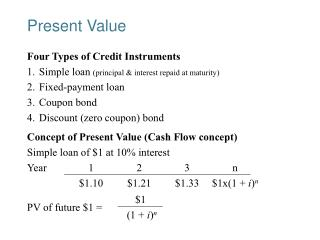DownloadDownload PresentationPresent Value

# Present Value

Télécharger la présentation## Present Value

- - - - - - - - - - - - - - - - - - - - - - - - - - - E N D - - - - - - - - - - - - - - - - - - - - - - - - - - -
##### Presentation Transcript

1. Present Value Four Types of Credit Instruments 1. Simple loan (principal & interest repaid at maturity) 2. Fixed-payment loan 3. Coupon bond 4. Discount (zero coupon) bond Concept of Present Value (Cash Flow concept) Simple loan of \$1 at 10% interest Year 1 2 3 n \$1.10 \$1.21 \$1.33 \$1x(1 + i)n \$1 PV of future \$1 = (1 + i)n

2. Yield to Maturity: Loans Yield to maturity = interest rate that equates today’s value with present value of all future payments (YTM representation of interest rate) What is the “today’s value”? 1. Simple Loan (i = 10%) \$100 = \$110/(1 + i)  \$110 – \$100 \$10 i = = = 0.10 = 10% \$100 \$100 2. Fixed Payment Loan (i = 12%) \$126 \$126 \$126 \$126 \$1000 = + + + ... + (1+i) (1+i)2 (1+i)3 (1+i)25 FPFPFPFP LV = + + + ... + (1+i) (1+i)2 (1+i)3 (1+i)n LV – loan value, FP – fixed payment

3. Yield to Maturity: Bonds 3. Coupon Bond (Coupon rate = 10% = C/F) , C – coupon payment, F – face value, P - price \$100 \$100 \$100 \$100 \$1000 P = + + + ... + + (1+i) (1+i)2 (1+i)3 (1+i)10 (1+i)10 CCCCF P = + + + ... + + (1+i) (1+i)2 (1+i)3 (1+i)n (1+i)n Consol: Fixed coupon payments of \$C forever CC P = i = i P 4. Discount Bond (P = \$900, F = \$1000), one year \$1000 \$900 = (1+i) \$1000 – \$900 i = = 0.111 = 11.1% \$900 F – P i = P 

4. Relationship Between Price and Yield to Maturity Three Interesting Facts in Table 1 1. When bond is at par, yield equals coupon rate 2. Price and yield are negatively related 3. Yield greater than coupon rate when bond price is below par value

5. Current Yield C ic = P Two Characteristics 1. Is better approximation to yield to maturity, nearer price is to par and longer is maturity of bond 2. Change in current yield always signals change in same direction as yield to maturity Yield on a Discount Basis (F – P) 360 idb = x F (number of days to maturity) One year bill, P= \$900, F = \$1000 \$1000 – \$900 360 idb = x = 0.099 = 9.9% \$1000 365 Two Characteristics 1. Understates yield to maturity; longer the maturity, greater is understatement 2. Change in discount yield always signals change in same direction as yield to maturity

6. Bond Page of the Newspaper

7. Distinction Between Interest Rates and Returns Rate of Return C+ Pt+1 – Pt RET = = ic + g Pt C where: ic = = current yield Pt Pt+1 – Pt g = = capital gain Pt

8. Maturity and the Volatility of Bond Returns Key Findings from Table 2 1. Only bond whose return = yield is one with maturity = holding period 2. For bonds with maturity > holding period, i P implying capital loss 3. Longer is maturity, greater is % price change associated with interest rate change 4. Longer is maturity, more return changes with change in interest rate 5. Bond with high initial interest rate can still have negative return if i Conclusion from Table 2 Analysis 1. Prices and returns more volatile for long-term bonds because have higher interest-rate risk 2. No interest-rate risk for any bond whose maturity equals holding period

9. Distinction Between Real and Nominal Interest Rates Real Interest Rate Interest rate that is adjusted for expected changes in the price level ir = i – e 1. Real interest rate more accurately reflects true cost of borrowing 2. When real rate is low, greater incentives to borrow and less to lend if i = 5% and e = 3% then: ir = 5% – 3% = 2% if i = 8% and e = 10% then ir = 8% – 10% = –2% However, more useful in practice is the after-tax real interest rate: ir = i(1-) – e ecan be obtained from TIPS (inflation indexed bonds)

10. U.S. Real and Nominal Interest Rates